## Acceleration and Retardation

Subject: Science

#### Overview

Acceleration is defined as the change in velocity per unit time. The negative acceleration is called retardation or deceleration. This note provides us with an information about acceleration and retardation.

#### AccelerationAcceleration is defined as the change in velocity per unit time. Acceleration is measured in m/s2.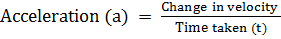=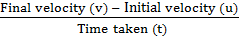Retardation

The negative acceleration is called retardation or deceleration. Its unit is same as acceleration.

Example:

1. Car starts from rest. After 5 seconds, its velocity becomes 10 m/s. Then find its acceleration.

Initial velocity (u) = 0

Final velocity (v) = 10 m/s

Time taken (t) = 5 s

Acceleration (a) =?

Or,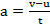Or,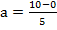∴ a = 2m/s2

2. The Car which starts from the rest has an acceleration of 0.3 m/s2. After 1 minute what will be its acceleration? What is the distance travelled by car?

Initial velocity (u) = 0

Time taken (t) = 1 min= 1 × 60 s = 60 s

Acceleration (a) = 0.3 m/s2

Final velocity (v) =?

Distanced travelled (s) =?

According to formula, v= u+ at

Or, v = 0 + 0.3 × 60

V= 18 m/s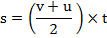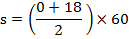∴ s =540 m

Equation of motion of uniform accelerationEquations involving displacement, initial velocity, final velocity, acceleration and time of the motion of a moving body are equations of motion.

Considering a body moving in a straight line with uniform acceleration is shown in the figure.

Let,

Displacement = s

Initial velocity = u

Final velocity = v

Acceleration = a

Time taken = t

Relation between u, v, a and t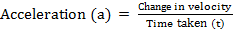Or,Or, at = v-u

∴ v= u + at ………….. (i)

This is the first equation of motion.

Relation between u,s, v and t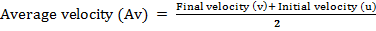Or,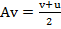Or, $\text{Average Velocity}=\frac{\text{Total Displacement(s)}}{\text{Total Time Taken(t)}}$

Or,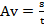As both, equation is equal

Or,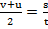Or, 2s = (u + v)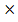t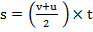……………… (ii)

This is the second equation of the motion.

Relation between s, u, a and t

V= u + at………….. (i)

s = (u + v)t…………… (ii)

Putting value of v from equation (i) in (ii)

Or,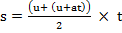Or, s =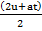Or, s = 2u×t + at × t× ½

∴ s = ut + ½ at2………………. (iii)

This is the third equation of motion.

##### Things to remember
• Acceleration is defined as the change in velocity per unit time. Acceleration is measured in m/s2.
• The negative acceleration is called retardation or deceleration. Its unit is same as acceleration.
• Equations involving displacement, initial velocity, final velocity, acceleration and time of motion of a moving body are equations of motion.

• It includes every relationship which established among the people.
• There can be more than one community in a society. Community smaller than society.
• It is a network of social relationships which cannot see or touched.
• common interests and common objectives are not necessary for society.
##### Videos for Acceleration and Retardation

Acceleration is defined as the change in velocity per unit time. Acceleration is measured in m/s2.

Solution

Initial velocity (u) = 0

Final velocity (v) = 10 m/s

Time taken (t) = 5 s

Acceleration (a) =?

Or,Or,∴ a = 2m/s2

Solution:

Initial velocity (u) = 0

Time taken (t) = 1 min= 1 × 60 s = 60 s

Acceleration (a) = 0.3 m/s2

Final velocity (v) =?

Distanced travelled (s) =?

According to formula, v= u+ at

Or, v = 0 + 0.3 × 60

V= 18 m/s∴ s =540 m

Solution:Or,Or, at = v-u

∴ v= u + at ………….. (i)

This is the first equation of motion.Or,Or,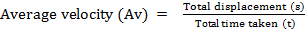Or,As both, equation is equal

Or,Or, 2s = (u + v)t……………… (ii)

This is the second equation of the motion.

Solution:

V= u + at………….. (i)

s = (u + v)t…………… (ii)

Putting value of v from equation (i) in (ii)

Or,Or, s =Or, s = 2u×t + at × t× ½

∴ s = ut + ½ at2………………. (iii)

This is the third equation of motion.